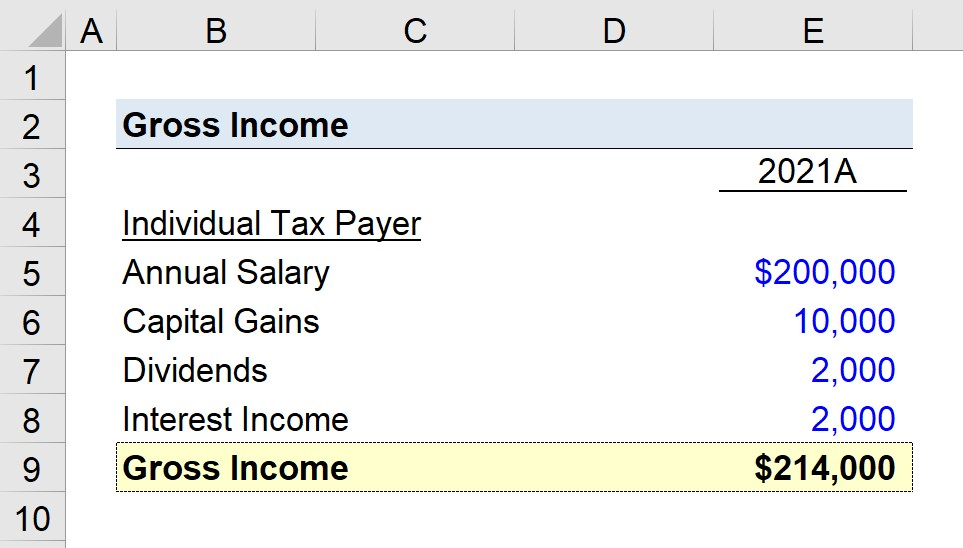Welcome to Wall Street Prep! Use code at checkout for 15% off.# Gross Income

Guide to Understanding Gross Income## How to Calculate Gross Income (Step-by-Step)

Gross income is defined as the total amount of income earned by an individual before taxes or any applicable deductions.

Therefore, the gross income is equal to the sum of an individual’s total earned income, less any tax-exempt sources of income.

The gross income is inclusive of sources such as the following:

With that said, the gross income of an individual is the starting point from which the taxable income is calculated.

For most individuals, wages will comprise the majority of one’s total earnings, but other side income must also be accounted for, such as the interest income from investments (e.g. savings accounts and the realized gains from stocks).

## Tax-Exempt Sources of Income

While sources of income such as salary received from an employer are subject to taxation, other forms of income are either partially or entirely exempt from being taxed such as:

• Inheritance
• Municipal Bonds
• Workers’ Compensation
• Proceeds from Life Insurance
• Health Benefits
• Voluntary Benefits
• Roth IRA Contributions

The tax status of each item is determined by the Internal Revenue Code (IRC), i.e. the “IRS tax code”.

## Gross Income Formula

The formula for calculating gross income is as follows.

Gross Income = Σ Income Earned

For companies, gross income – more often reported as “Gross Profit” – is calculated by subtracting the cost of goods sold (COGS) from its revenue for the year.

Gross Profit = RevenueCost of Goods Sold (COGS)
• Revenue: The amount of sales generated by the company from selling its products or services.
• Cost of Goods Sold: COGS are the expenses incurred that are directly tied to its revenue model such as the cost of material and the cost of direct labor.

The lower the company’s COGS, the higher its gross profit and gross margin (and vice versa).

## What is Adjusted Gross Income (AGI)?

To calculate adjusted gross income, the gross earnings figure is adjusted for any deductions or exemptions, which are formally known as “above-the-line” deductions.

After subtracting the appropriate amount with guidance from a professional accountant, the post-deduction amount results in adjusted gross income (AGI).

However, the amount the individual owes in taxes is neither based on the non-adjusted nor the adjusted gross figure.

Instead, the adjusted gross income (AGI) metric must be adjusted for the standard deduction or itemized deductions, which then results in the individual’s taxable income for the given year.

The reduction amount is the greater of either the:

• Standard Deduction (or)
• Itemized Deduction

## Gross vs. Net Income: What is the Difference?

The gross income of an individual represents the total earnings a person receives in the taxable year before taxes and any deductions are considered.

Once taxes and deductions are deducted, the remaining amount is net income.

Net income is the leftover amount of money belonging to the individual.

The percentage difference between gross income and net income tends to be more predictable for individuals rather than companies because the two values can vary substantially more and fluctuate year-to-year for companies due to the increased number of variables that can impact a company’s profitability.

If the variance between a company’s gross profit and net income is substantial, that may mean that the company needs to reconsider its spending habits and implement cost-cutting measures to improve its profitability, i.e. eliminate or reduce unnecessary costs.

## Gross Income Calculator – Excel Template

We’ll now move on to a modeling exercise, which you can access by filling out the form below.Submitting...

## Individual Taxpayer Gross Income Calculation Example

Suppose an individual has begun the process of filing their taxes with the IRS for fiscal year 2021.

In 2021, the individual’s annual salary was \$200,000, along with three other sources of income:

• Capital Gains = \$10,000
• Dividends = \$2,000
• Interest Income = \$2,000

The total dollar value of the realized capital gains from stocks was \$10,000, along with \$2,000 in dividends received as a shareholder and \$2,000 in interest income from other investments.

After calculating the sum of all four sources of income, we arrive at a gross income figure of \$214,000 for the individual in 2021.

• Gross Income = \$200,000 + \$10,000 + \$2,000 + \$2,000 = \$214,000Step-by-Step Online Course

### Everything You Need To Master Financial Modeling

Enroll in The Premium Package: Learn Financial Statement Modeling, DCF, M&A, LBO and Comps. The same training program used at top investment banks.

Inline Feedbacks